Home | ARTS | Operations Management | Problem of Earliest And Latest Times

# Problem of Earliest And Latest Times

Posted On :  24.06.2018 12:34 am

The following details are available regarding a project:

Problem

The following details are available regarding a project:Determine the earliest and latest times, the total float for each activity, the critical activities and the project completion time.

Solution

With the given data, we construct the following network diagram for the project.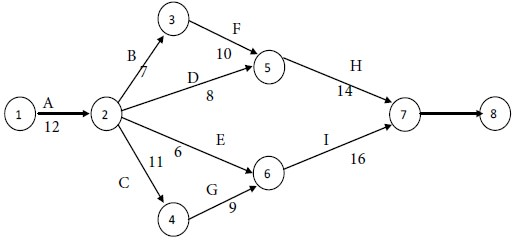Consider the paths, beginning with the start node and stopping with the end node. There are four such paths for the given project. They are as follows: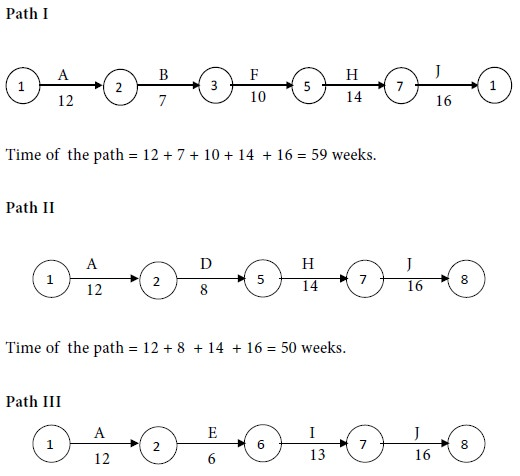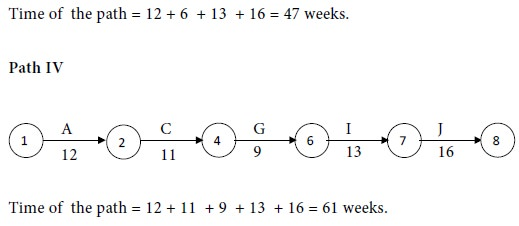Compare the times for the four paths. Maximum of {51, 50, 47, 61} = 61.

We see that the maximum time of a path is 61 weeks.

Forward pass

Calculation of Earliest Expected Time of Occurrence of EventsUsing the above values, we obtain the Earliest Start Times of the activities as follows:Backward pass

Calculation of Latest Allowable Time of Occurrence of Events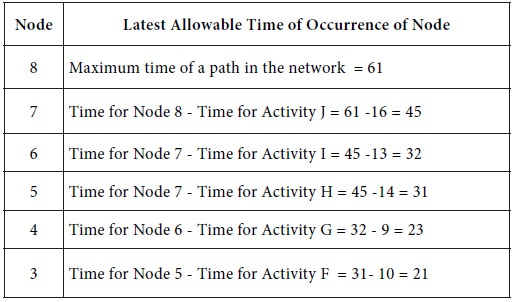Using the above values, we obtain the Latest Finish Times of the activities as follows: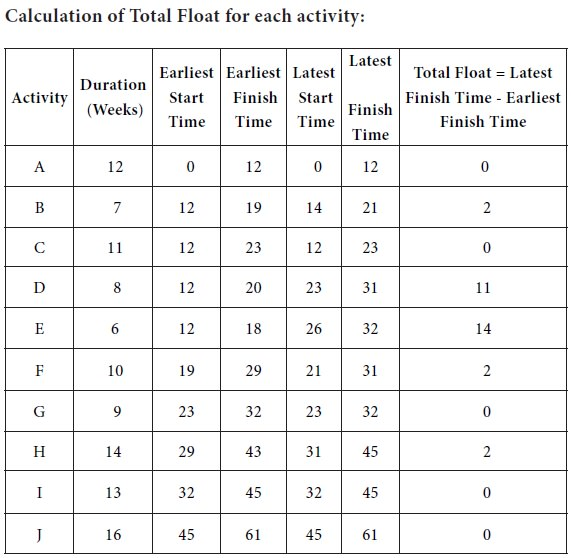The activities with total float = 0 are A, C, G, I and J. They are the critical activities.

Project completion time = 61 weeks.

Problem 2

The following are the details of the activities in a project: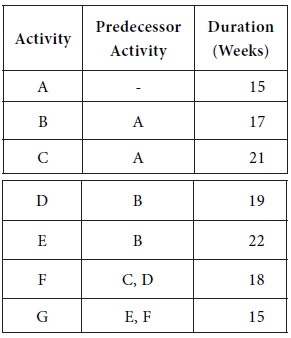Calculate the earliest and latest times, the total float for each activity and the project completion time.

Solution

The following network diagram is obtained for the given project.Consider the paths, beginning with the start node and stopping with the end node. There are three such paths for the given project. They are as follows:Compare the times for the three paths. Maximum of {69, 84, 69} = 84. We see that the maximum time of a path is 84 weeks.

Forward pass

Calculation of Earliest Time of Occurrence of Events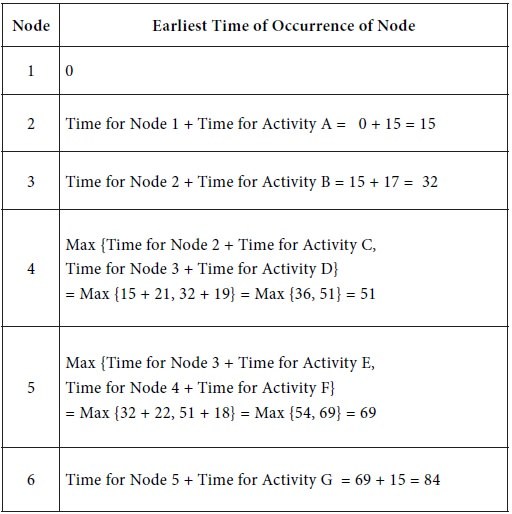Backward pass

Calculation of the Latest Allowable Time of Occurrence of Events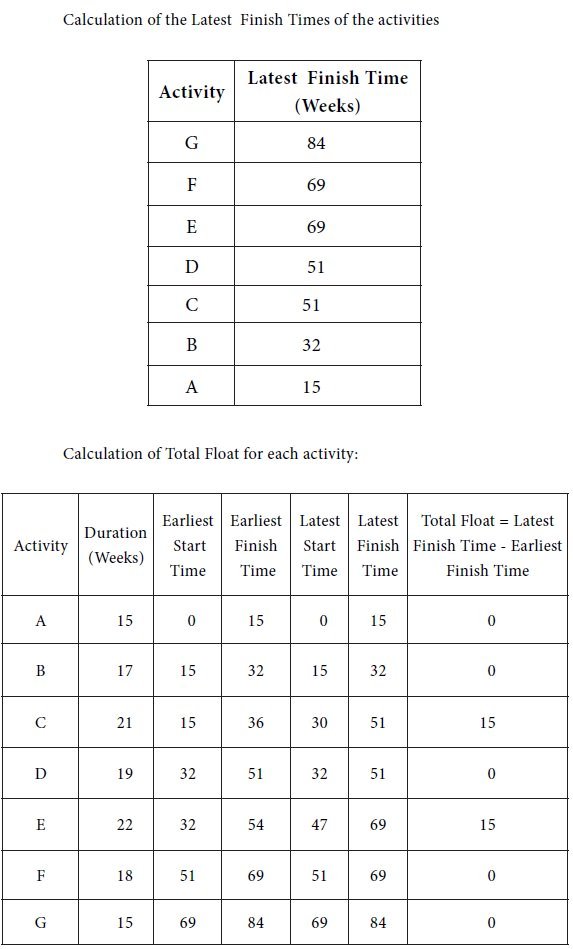The activities with total float = 0 are A, B, D, F and G. They are the critical activities.

Project completion time = 84 weeks.

Problem 3

Consider a project with the following details: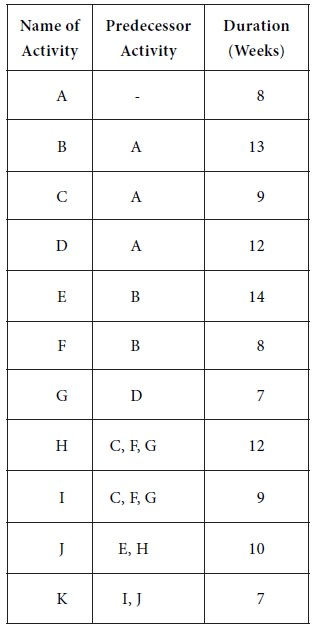Determine the earliest and latest times, the total float for each activity, the critical activities, the slacks of the events and the project completion time.

Solution

The following network diagram is got for the given project: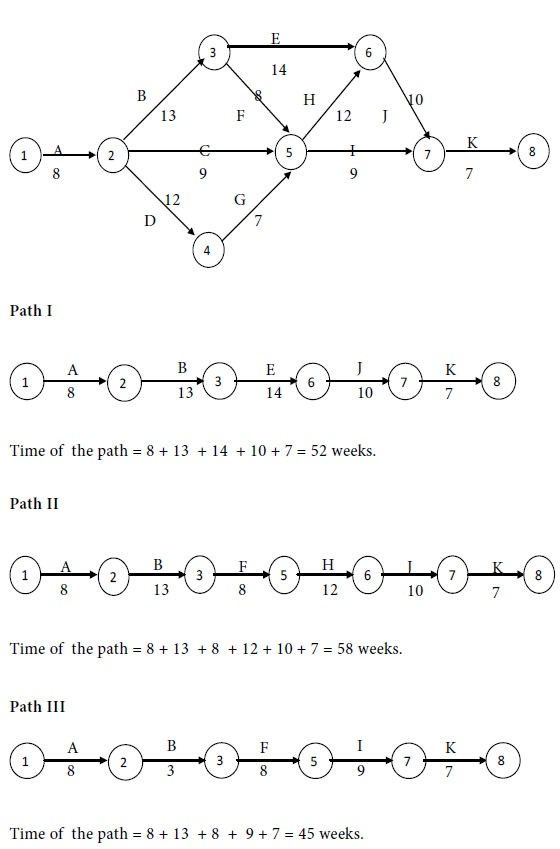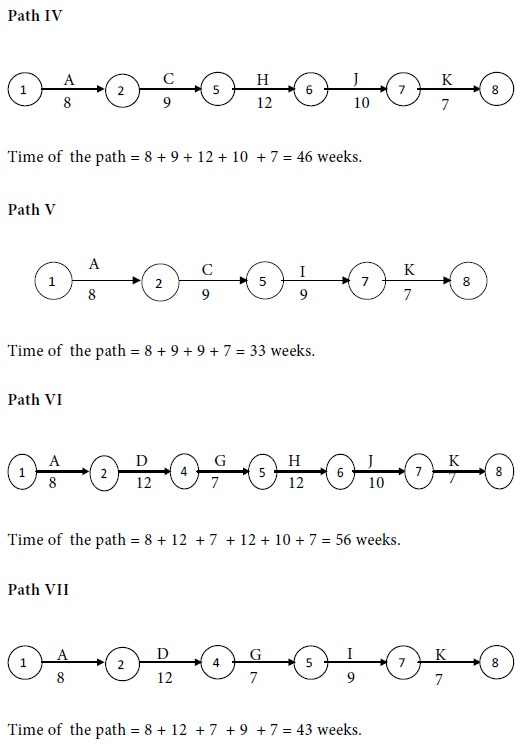Compare the times for the three paths. Maximum of {52, 58, 45, 46, 33, 56, 43} = 58.

We see that the maximum time of a path is 58 weeks.

Forward pass

Calculation of Earliest Time of Occurrence of Events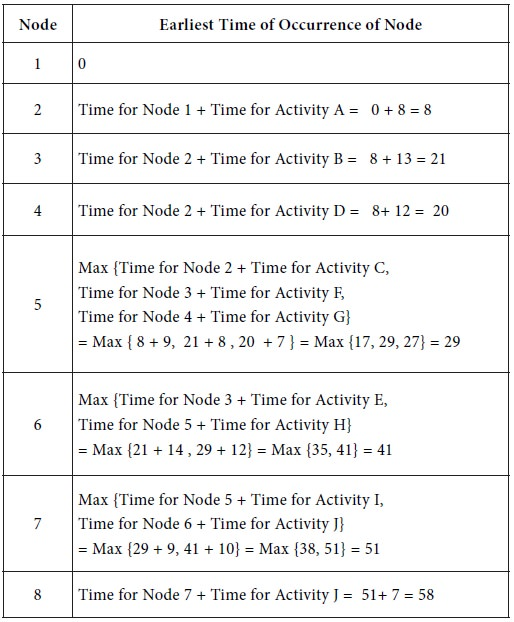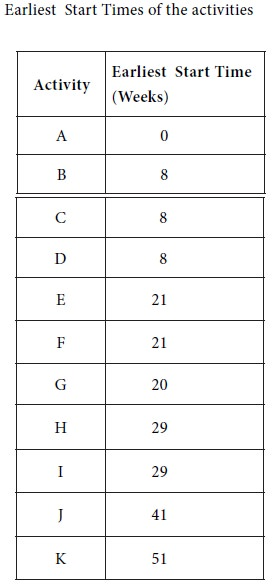Backward pass

Calculation of Latest Allowable Time of Occurrence of Events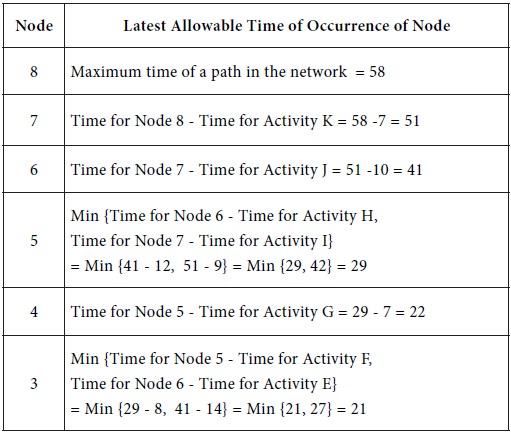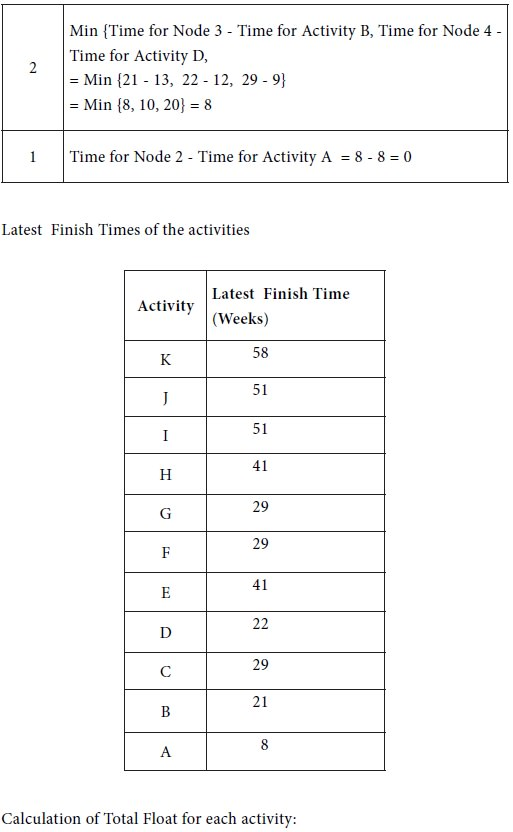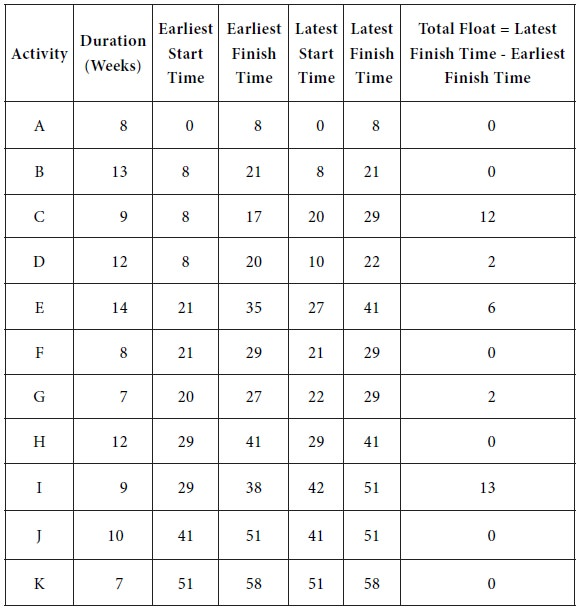The activities with total float = 0 are A, B, F, H, J and K. They are the critical activities.

Project completion time = 58 weeks.

Calculation of slacks of the events

Slack of an event = Latest Allowable Time of Occurrence of the event - Earliest Expected Time of Occurrence of that event.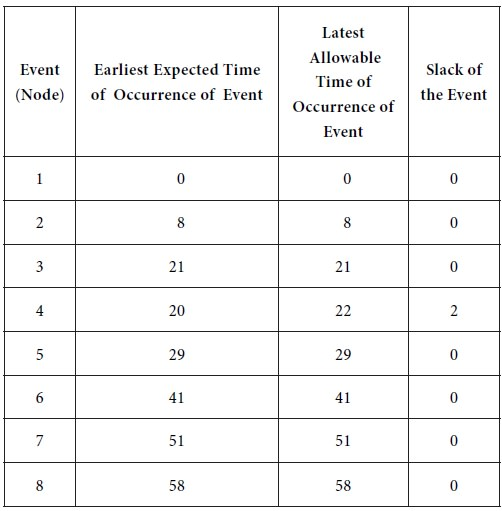Interpretation

On the basis of the slacks of the events, it is concluded that the occurrence of event 4 may be delayed upto a maximum period of 2 weeks while no other event cannot be delayed.

Tags : Operations Management - Network Problems
Last 30 days 3981 views